Need help? Email help@shout.education

# Reactions of Acid Anhydrides With Ammonia or Primary Amines

This page looks at the reactions of acid anhydrides with ammonia and with primary amines. These reactions are considered together because their chemistry is so similar.

There is also a great similarity between acid anhydrides and acyl chlorides (acid chlorides) as far as these reactions are concerned. Concentrate on these similarities as you go through this page because it should help you to remember.

## Similarities Between the Reactions

### Comparing the Structures of Ammonia and Primary Amines

Each substance contains an -NH2 group. In ammonia, this is attached to a hydrogen atom. In a primary amine, it is attached to an alkyl group (shown by "R" in the diagram below) or a benzene ring.

Note: If you aren't sure about using this symbol for a benzene ring, you could follow this link to find out all about it. It is likely to take you some time, though, and you may have to visit several other pages as well.

It isn't particularly important in the context of the current page. All you need to know is that at each corner of the hexagon there is a carbon atom, together with a hydrogen atom apart from where the -NH2 group is attached.

### Comparing the Reactions of Acyl Chlorides and Acid Anhydrides With These Compounds

Because the formula is much easier, it helps to start with the acyl chlorides.

#### The reactions with acyl chlorides

We'll take ethanoyl chloride as typical of the acyl chlorides.

Taking a general case of a reaction between ethanoyl chloride and a compound XNH2 (where X is hydrogen, or an alkyl group, or a benzene ring). The reaction happens in two stages:

##### First: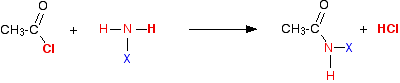So in each case, you initially get hydrogen chloride gas – the hydrogen coming from the -NH2 group, and the chlorine from the ethanoyl chloride. Everything left over just gets joined together.

But ammonia and amines are basic, and react with the hydrogen chloride to produce a salt. So the second stage of the reaction is:

\text{XNH}_2 + \text{HCl} \longrightarrow {\text{XNH}_3}^+\text{Cl}^-

#### The same reactions with acid anhydrides

Ethanoic anhydride is the only one you are likely to come across for UK A-level purposes.

Again, the reaction happens in two stages. In the first: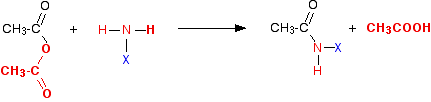If you compare this with the acyl chloride equation, you can see that the only difference is that ethanoic acid is produced as the second product of the reaction rather than hydrogen chloride.

Note: The colour coding in these equations is to try to help you to see where everything ends up and where it came from, and to enable you to compare the two reactions more easily. You can think of the entire bit of the ethanoic anhydride shown in red as being exactly the equivalent of the chlorine atom in the acyl chloride as far as these reactions are concerned.

Then the ethanoic acid reacts with excess ammonia or amine to give a salt – this time an ethanoate.

\text{CH}_3\text{COOH} + \text{XNH}_2 \longrightarrow \text{CH}_3\text{COO}^-{}^+\text{NH}_3\text{X}

This looks more difficult than the acyl chloride case because of the way the salt is written. You get an ethanoate ion and a positive ion with this structure: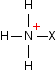This is easier to understand with real compounds – as you will see below.

These reactions are just the same as the corresponding acyl chloride reactions except:

• Initially, ethanoic acid is formed as the second product rather than hydrogen chloride gas.
• The second stage of the reaction involves the formation of an ethanoate rather than a chloride.
• The reactions are slower. Acid anhydrides aren't so violently reactive as acyl chlorides, and the reactions normally need heating.

## The Individual Reactions

### The Reaction With Ammonia

In this case, the "X" in the equations above is a hydrogen atom. So in the first instance you get ethanoic acid and an organic compound called an amide.

Amides contain the group -CONH2. In the reaction between ethanoic anhydride and ammonia, the amide formed is called ethanamide.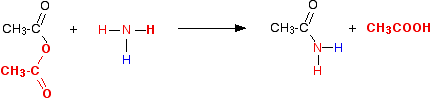This is more usually (and more easily!) written as:

(\text{CH}_3\text{CO})_2\text{O} + \text{NH}_3 \longrightarrow \underset{\color{#467abf}{\text{ethanamide}}}{\text{CH}_3\text{CONH}_2} +~\text{CH}_3\text{COOH}

The ethanoic acid produced reacts with excess ammonia to give ammonium ethanoate.

\text{CH}_3\text{COOH} + \text{NH}_3 \longrightarrow \underset{\color{#467abf}{\text{ammonium ethanoate}}}{\text{CH}_3\text{COO}^-{}^+\text{NH}_4}

and you can combine all this together to give one overall equation:

(\text{CH}_3\text{CO})_2\text{O} + 2\text{NH}_3 \longrightarrow \underset{\color{#467abf}{\text{ethanamide}}}{\text{CH}_3\text{CONH}_2} + \underset{\color{#467abf}{\text{ammonium ethanoate}}}{\text{CH}_3\text{COO}^-{}^+\text{NH}_4}

You need to follow this through really carefully, because the two products of the reaction overall can look confusingly similar.

The corresponding reaction with an acyl chloride is:

\text{CH}_3\text{COCl} + 2\text{NH}_3 \longrightarrow \text{CH}_3\text{CONH}_2 + {\text{NH}_4}^+\text{Cl}^-

Note: There isn't anything difficult involved in the fact that I have moved the position of the positive charge in the ammonium ion in this equation compared with the previous one.In each case I have placed it as close as possible to the negative charge in the ethanoate ion or chloride ion.

In the ethanoate case, that makes it easier to draw the structure of the similar positive ion in the amine equations below.

### The Reaction With Primary Amines

#### The reaction with methylamine

We'll take methylamine as typical of primary amines where the -NH2 is attached to an alkyl group.

The initial equation would be: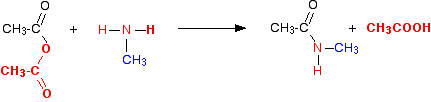The first product this time is called an N-substituted amide.

If you compare the structure with the amide produced in the reaction with ammonia, the only difference is that one of the hydrogens on the nitrogen has been substituted for a methyl group.

This particular compound is N-methylethanamide. The "N" simply shows that the substitution is on the nitrogen atom, and not elsewhere in the molecule.

The equation would normally be written:

(\text{CH}_3\text{CO})_2\text{O} + \text{CH}_3\text{NH}_2 \longrightarrow \underset{\color{#467abf}{\text{N-methylethanamide}}}{\text{CH}_3\text{CONHCH}_3} +~\text{CH}_3\text{COOH}

You can think of primary amines as just being modified ammonia. If ammonia is basic and forms a salt with the ethanoic acid, excess methylamine will do exactly the same thing.

\text{CH}_3\text{COOH} + \text{CH}_3\text{NH}_2 \longrightarrow \underset{\color{#467abf}{\text{methylammonium ethanoate}}}{\text{CH}_3\text{COO}^-{}^+\text{NH}_3\text{CH}_3}

The salt is called methylammonium ethanoate. It is just like ammonium ethanoate, except that one of the hydrogens has been replaced by a methyl group.

You would usually combine these equations into one overall equation for the reaction:

(\text{CH}_3\text{CO})_2\text{O} + 2\text{CH}_3\text{NH}_2 \longrightarrow \text{CH}_3\text{CONHCH}_3 + \text{CH}_3\text{COO}^-{}^+\text{NH}_3\text{CH}_3

The corresponding reaction with an acyl chloride is:

\text{CH}_3\text{COCl} + 2\text{CH}_3\text{NH}_2 \longrightarrow \text{CH}_3\text{CONHCH}_3 + {\text{CH}_3\text{NH}_3}^+\text{Cl}^-

#### The reaction with phenylamine (aniline)

Phenylamine is the simplest primary amine where the -NH2 group is attached directly to a benzene ring. Its old name is aniline.

In phenylamine, there isn't anything else attached to the ring as well. You can write the formula of phenylamine as C6H5NH2.

There is no essential difference between this reaction and the reaction with methylamine, but I just want to look at the structure of the N-substituted amide formed.

The overall equation for the reaction is:

(\text{CH}_3\text{CO})_2\text{O} + 2\text{C}_6\text{H}_5\text{NH}_2 \longrightarrow \text{CH}_3\text{CONHC}_6\text{H}_5 + \text{CH}_3\text{COO}^-{}^+\text{NH}_3\text{C}_6\text{H}_5

The products are N-phenylethanamide and phenylammonium ethanoate.

This reaction can sometimes look (even more!) confusing if the phenylamine is drawn showing the benzene ring, and especially if the reaction is looked at from the point of view of the phenylamine.

For example, the product molecule might be drawn looking like this: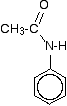If you stop and think about it, this is obviously the same molecule as in the equation above, but it stresses the phenylamine part of it much more.

Looking at it this way, notice that one of the hydrogens of the -NH2 group has been replaced by an acyl group – an alkyl group attached to a carbon-oxygen double bond.

You can say that the phenylamine has been acylated or has undergone acylation.

Because of the nature of this particular acyl group, it is also described as ethanoylation. The hydrogen is being replaced by an ethanoyl group, CH3CO-.

Note: For UK A-level purposes, this is probably the most likely context that you will meet this particular reaction in. You would need to know that phenylamine reacted with ethanoic anhydride to produce an N-substituted amide, and that this was an example of acylation (or ethanoylation). You would probably be expected to draw the structure, but would be pretty unlucky if you had to write equations as above.